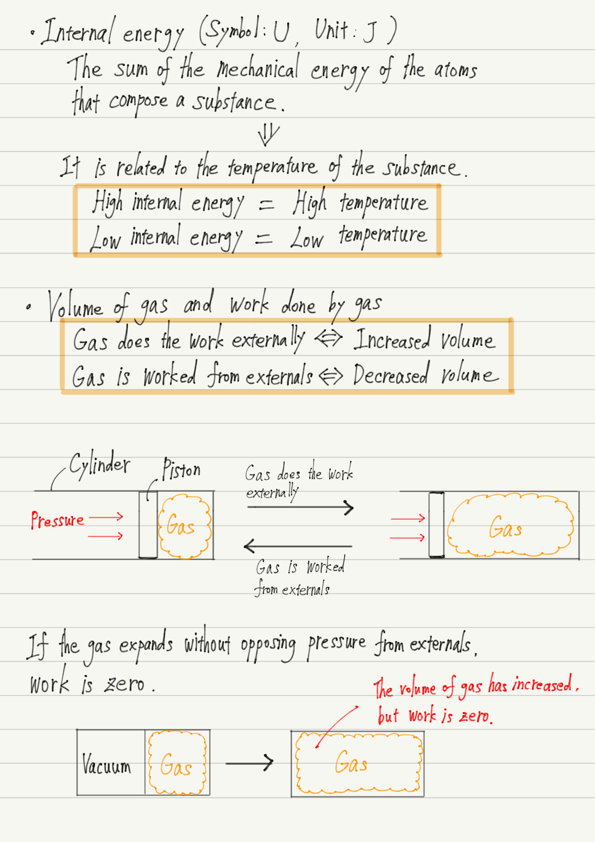# First Law of Thermodynamics(1)

Just as there is a fundamental law in mechanics called Newton’s laws of motion, there is a fundamental law in thermodynamics called the laws of thermodynamics.

Newton’s laws of motion are of three types, from the first to the third, but the laws of thermodynamics are of four types, from the zero to the third.
Two of these laws will be covered in this series of lectures: the first law and the second law.
In this and the next lecture, I would like to introduce the first law.

## Before learning the first law of thermodynamics

Before introducing the law, some terminology must be explained.
This is because this law represents a formula that cannot be used by memorizing only the formula!

If you do not understand the meaning of the letters used in the formulas, you will not be able to make the correct substitutions when you are ready to calculate.

There are three letters in the first law of thermodynamics: Q, U, and W. Let’s look at what each of these three letters represents, one at a time.

## ① Q

First, Q. This has appeared before.
Yes, this is heat quantity. To be more precise, here it is the heat quantity “given” to an object.

## ② U

U is what we call the internal energy of an object.
Internal energy is a term that appears for the first time, but we will discuss it in more detail another time.

There are two things you should know about internal energy at this lecture.

・Internal energy of an object = the sum of the mechanical energy possessed by the atoms and molecules that make up the object.

・The internal energy is closely related to the temperature of the object, and the higher the temperature of the object, the greater the internal energy.

## ③ W

Finally, W. This represents the work that also appeared in mechanics.
It is precisely “the work the gas did externally” since the gas is the main actor in the first law of thermodynamics.

Don’t just hear the words and think you understand, okay?
What exactly do you mean by “gas does the work”?

“Work” means, in mechanics, “moving an object by acting a force”, but in thermodynamics, it means “expanding a gas against an external force(i.e., increasing its volume)”.

Conversely, when the volume of a gas is reduced by an external force, it is expressed in the passive voice as “the gas is worked from the externals”.

Pay attention to the use of words and what they represent.

## Summary of this lecture## Next Time

In next lecture, I will explain what is in the first law of thermodynamics!

https://www.yukimura-physics.com/entry/therm-f08

error: Content is protected !!